## RD Sharma Class 8 Solutions Chapter 9 Linear Equations in One Variable Ex 9.1

These Solutions are part of RD Sharma Class 8 Solutions. Here we have given RD Sharma Class 8 Solutions Linear Equations in One Variable Ex 9.1

Other Exercises

Solve each of the following equations and also verify your solution :
Question 1.
9$$\frac { 1 }{ 4 }$$ = y – 1$$\frac { 1 }{ 3 }$$
Solution: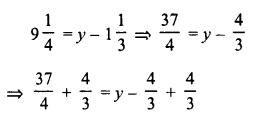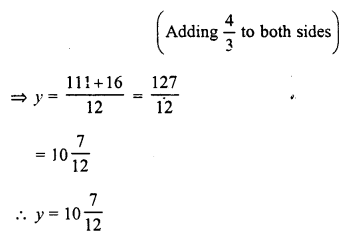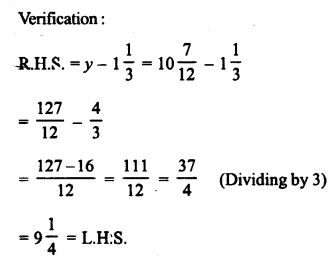Question 2.
$$\frac { 5x }{ 3 }$$ + $$\frac { 2 }{ 5 }$$ = 1
Solution: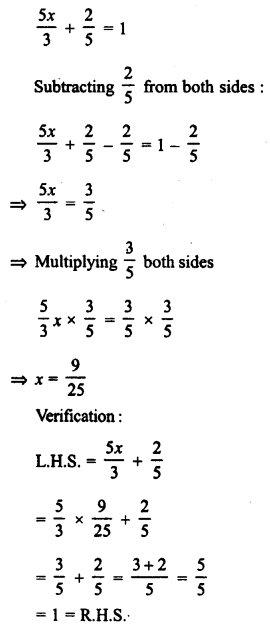Question 3.
$$\frac { x }{ 2 }$$ + $$\frac { x }{ 3 }$$ + $$\frac { x }{ 4 }$$ = 13
Solution: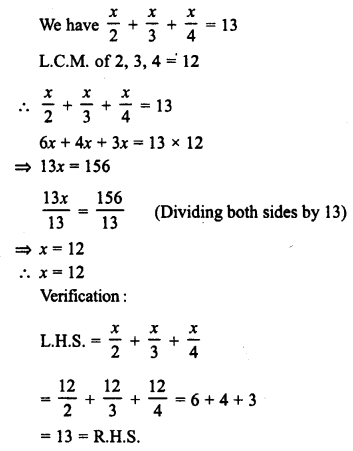Question 4.
$$\frac { x }{ 2 }$$ + $$\frac { x }{ 8 }$$ = $$\frac { 1 }{ 8 }$$
Solution: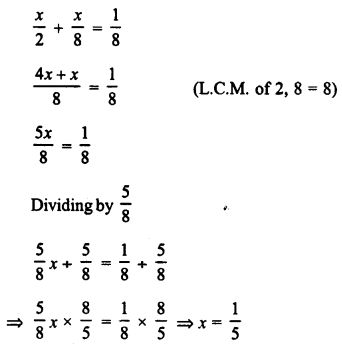Question 5.
$$\frac { 2x }{ 3 }$$ – $$\frac { 3x }{ 8 }$$ = $$\frac { 7 }{ 12 }$$
Solution: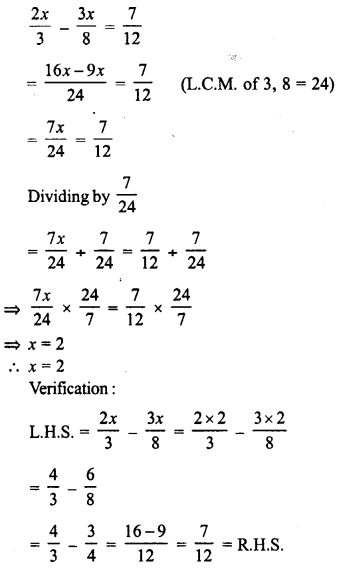Question 6.
(x + 2) (x + 3) + (x – 3) (x – 2) – 2x (x + 1) = 0
Solution:
(x + 2) (x + 3) + (x – 3) (x – 2) – 2x (x + 1) = 0
⇒ [x² + (2 + 3) x + 2 x 3] + [x² + (-3 – 2) x + (-3) (-2)] – 2x² – 2x = 0
⇒ x² + 5x + 6 + x² – 5x + 6 – 2x² – 2x = 0
⇒ x² + x² – 2x² + 5x – 5x – 2x + 6 + 6 = 0
⇒ -2x + 12 = 0
Subtracting 12 from both sides,
-2x + 12 – 12 = 0 – 12
⇒ -2x = -12
Dividing by -2,
x = 6
Verification:
L.H.S. = (x + 2) (x + 3) + (x – 3) (x – 2) – 2x (x + 1)
= (6 + 2) (6 + 3) + (6 – 3) (6 – 2) – 2 x 6 (6 + 1)
= 8 x 9 + 3 x 4 – 12 x 7
= 72 + 12 – 84
= 84 – 84
= 0
= R.H.S.

Question 7.
$$\frac { x }{ 2 }$$ – $$\frac { 4 }{ 5 }$$ + $$\frac { x }{ 5 }$$ +$$\frac { 3x }{ 10 }$$ = $$\frac { 1 }{ 5 }$$
Solution: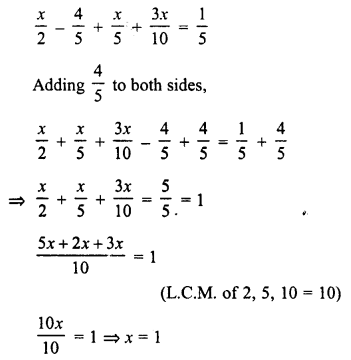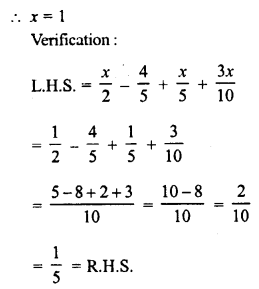Question 8.
$$\frac { 7 }{ x }$$ + 35 = $$\frac { 1 }{ 10 }$$
Solution: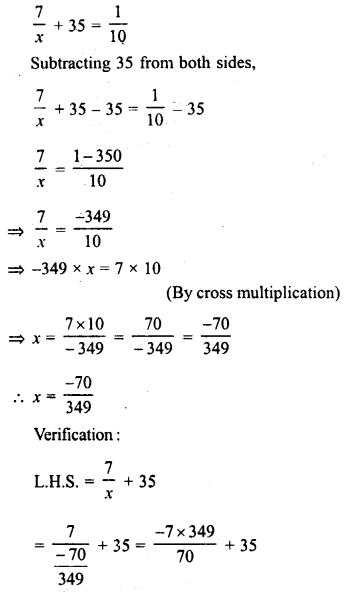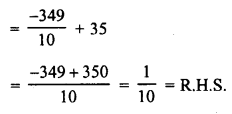Question 9.
$$\frac { 2x – 1 }{ 3 }$$ – $$\frac { 6x – 2 }{ 5 }$$ = $$\frac { 1 }{ 3 }$$
Solution: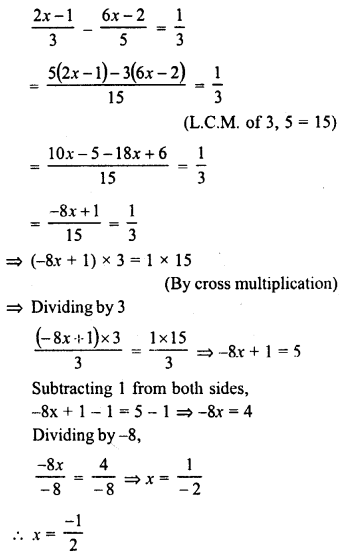Question 10.
13 (y – 4) – 3 (y – 9) – 5 (y + 4) = 0
Solution:
13 (y – 4) – 3 (y – 9) – 5 (y + 4) = 0
⇒ 13y – 52 – 3y + 27 – 5y – 20 = 0
⇒ 13y – 3y – 5y – 52 + 27 – 20 = 0
⇒ 13y – 8y – 72 + 27 = 0
⇒ 5y – 45 = 0
Dividing by 5,
y = 9
Verification:
L.H.S. = 13 (y – 4) – 3 (y – 9) – 5 (y + 4)
= 13 (9 – 4) – 3 (9 – 9) – 5 (9 + 4)
= 13 x 5 – 3 x 0 – 5 x 13
= 65 – 0 – 65
= 0
= R.H.S.

Question 11.
$$\frac { 2 }{ 3 }$$ (x – 5) – $$\frac { 1 }{ 4 }$$ (x – 2) = $$\frac { 9 }{ 2 }$$
Solution: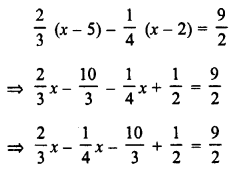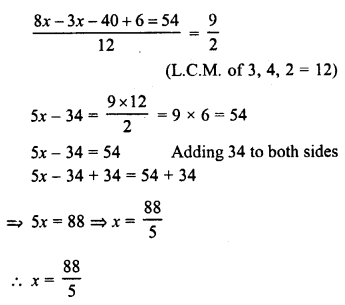Hope given RD Sharma Class 8 Solutions Linear Equations in One Variable Ex 9.1 are helpful to complete your math homework.

If you have any doubts, please comment below. Learn Insta try to provide online math tutoring for you.